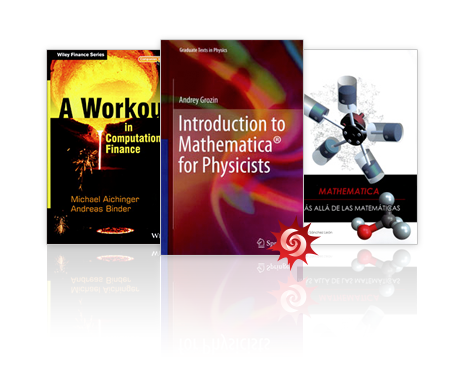Browse by Topic
Related Topics

# Read Up on Mathematica in Many Subjects

Researchers and professionals from around the world are frequently using Mathematica to further their fields of study. We like to recognize these published books and papers that incorporate our technologies and catalog them so that you can find resources for using Mathematica in subjects you need. Here are some recent publications:Introduction to Mathematica for Physicists
by A. Grozin
This book provides an introduction to using Mathematica specifically in the context of physics, chemistry, and mathematics. It gives an overview of the structure of the language used to make computations in Mathematica, and provides strategies and practice examples to illustrate how software can be used in scientific fields of research.

A Workout in Computational Finance
by Michael Aichinger, Andreas Binder
For students and professionals pursuing the finance industry, this book provides numerous algorithms and methods in finance, with supplemental interactive examples that can be downloaded in the Computable Document Format™ (CDF).

Information Processing Volume II: The Maximum Entropy Principle
by David J. Blower
This second volume introduces the Maximum Entropy Principle in the field of information processing, working with probability distributions and the mathematical expressions they are built upon. Also included in each chapter are examples and exercises specifically for practicing these concepts in Mathematica.

Komplexe Systeme—Neue Ansätze und zahlreiche Beispiele (German)
(Complex Systems—New Approaches and Numerous Examples)
by Frank Brand
This publication shows how to use Mathematica to model diverse complex systems as highly-connected graphs of components, and addresses relevant graph-theoretic topics such as path-finding and Hamiltonian cycles.

Mathématiques pour les sciences de l’ingénieur avec Mathematica
(French)
(Mathematics for the Engineering Sciences with Mathematica)
by A. Carmasol
This mathematics textbook is for engineering students. It tailors its lessons specifically around Mathematica, with exercises and examples included throughout the volume.

Mathematica, Más Allá de las Matemáticas (Spanish)
(Mathematica, Beyond Mathematics)
by J. Guillermo Sánchez León
This textbook takes a look at the modern principles and applications of mathematics, using Mathematica as a tool to visualize and explore them in a wide array of topics, from cosmology in astrophysics to stock quotes in finance.

Modelos Neuronales Aplicados en Economía, Casos Practicos mediante Mathematica (Spanish)
(Neural Models in Economics, with Case Studies in Mathematica)
by Salvador Torra Porras, Enric Monte Moreno
This textbook illustrates neural networks and models in economics and finance, going over both theory and step-by-step examples using Mathematica.

Learning Differential Equations and Solutions by Examples (Japanese)
by Ben Nohara
This differential equations textbook provides visualizations and examples in Mathematica for a variety of applied uses, including understanding whale migration and modeling ecological systems.

Introduction to Mathematics for Economics Students (Japanese)
by Yukari Shirota